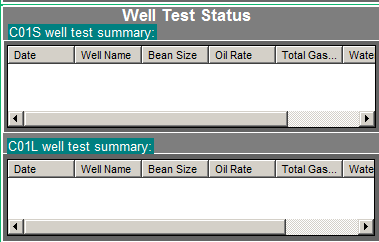# Extract Trend data with VBA?

Question asked by Timur on Jan 27, 2017
Latest reply on Feb 3, 2017 by Timur

I have a several Traces with different Variables running on real time. I have plotted all these traces in Trend1. Trend1 displays last 30 days data from now.

The Traces are defined as below:

1. Trace1: String ID

2. Trace2: Bean size

3. Trace3: Oil rate

4. Trace4: Total Gas Rate

5. Trace5. Water Rate

6. Trace6. GOR

Then i have wrote a code lets say to select "String ID" and I need to develop code that can read a selected "String ID" from traces upon request and give all the respective other trace data ( Bean size, Oil Rate) correspondingly to that time in respective ListView.

The output data i have put it on ListView.

' To run the code once every Display opens:

Private Sub Display_Open()

Call GetTraceValuesOne(Trend1, 1, ListView1)

Call GetTraceValuesTwo(Trend1, 1, ListView2)

End Sub

Sub GetTraceValuesOne(p As Trend, i As Long, lv As ListView)

Dim myValue As Variant

Dim myOil As Variant

Dim myBS As Variant

Dim myTGAS As Variant

Dim k, m, h As Long

Dim j As Integer

Dim myWTR   As Variant

Dim myGOR   As Variant

Dim myTime As Variant

Dim myStatus As Variant

Dim li As ListItem

With lv

For k = .ColumnHeaders.Count To 1 Step -1

Next k

.View = lvwReport

For k = .ListItems.Count To 1 Step -1

' clear the list

.ListItems.Remove k

Next k

End With

For j = 1 To p.TraceCount

p.CurrentTrace = i

'String ID

myValue = p.GetTraceValue(j, myTime, myStatus)

If myValue = "C-01L" Then

p.CurrentTrace = 2  'Bean Size

myBS = p.GetTraceValue(j, myTime, myStatus)

p.CurrentTrace = 3  'Oil Rate

myOil = p.GetTraceValue(j, myTime, myStatus)

p.CurrentTrace = 4  'Total Gas Rate

myTGAS = p.GetTraceValue(j, myTime, myStatus)

p.CurrentTrace = 5  'Water Rate

myWTR = p.GetTraceValue(j, myTime, myStatus)

p.CurrentTrace = 6  'GOR

myGOR = p.GetTraceValue(j, myTime, myStatus)

Set li = lv.ListItems.Add(, , myTime)

li.SubItems(1) = myValue

li.SubItems(2) = myBS

li.SubItems(3) = myOil

li.SubItems(4) = myTGAS

li.SubItems(5) = myWTR

li.SubItems(6) = myGOR

End If

Next j

End Sub

' there is a second ListView to look thru for another "String ID" :

Sub GetTraceValuesTwo(t As Trend, i As Long, lv As ListView)

Dim myValue1 As Variant

Dim myOil As Variant

Dim myBS As Variant

Dim myTGAS As Variant

Dim k, m, h As Long

Dim q As Integer

Dim myWTR   As Variant

Dim myGOR   As Variant

Dim myTime As Variant

Dim myStatus As Variant

Dim li As ListItem

Dim cTim, dTim As Variant

Dim u, b As Variant

With lv

For k = .ColumnHeaders.Count To 1 Step -1

Next k

.View = lvwReport

For k = .ListItems.Count To 1 Step -1

' clear the list

.ListItems.Remove k

Next k

End With

For q = 1 To t.TraceCount

t.CurrentTrace = i

'String ID

myValue1 = t.GetTraceValue(q, myTime, myStatus)

If myValue1 = "C-01S" Then

t.CurrentTrace = 2  'Bean Size

myBS = t.GetTraceValue(q, myTime, myStatus)

t.CurrentTrace = 3  'Oil Rate

myOil = t.GetTraceValue(q, myTime, myStatus)

t.CurrentTrace = 4  'Total Gas Rate

myTGAS = t.GetTraceValue(q, myTime, myStatus)

t.CurrentTrace = 5  'Water Rate

myWTR = t.GetTraceValue(q, myTime, myStatus)

t.CurrentTrace = 6  'GOR

myGOR = t.GetTraceValue(q, myTime, myStatus)

Set li = lv.ListItems.Add(, , myTime)

li.SubItems(1) = myValue1

li.SubItems(2) = myBS

li.SubItems(3) = myOil

li.SubItems(4) = myTGAS

li.SubItems(5) = myWTR

li.SubItems(6) = myGOR

End If

Next q

End Sub

Output results for 2 different "String ID":Trend1 with different traces showing last 30 days data:P.S. i have tried to manipulate with putting q=1 to any arbitrary number... for small numbers it is showing figures but not complete data..If i put huge number then it says followings:

"number submitted was outside of acceptable range for the value"

So i cannot resolve it until now where is errors.